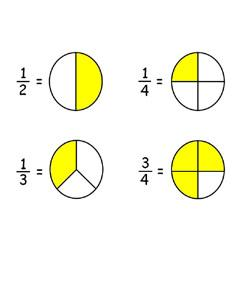# Dux Trial Test

11 Questions | Total Attempts: 886Settings• 1.
• 2.
1. Why did the narrator consult a dictionary that morning?
• A.

He wanted to find out the antonyms of the two words

• B.

He wanted to note down the definitions in his book

• C.

He wanted to find out the meanings of the two words

• D.

He did not have anything else to do that morning

• 3.
In the numeral 483 195, the value of the digit 8 is greaterthan the value of the digit 9 by __ .
• A.

7910

• B.

7991

• C.

79 910

• D.

79 991

• 4.
Add 2 795 to the product of 36 and 147. The answer is __ .
• A.

2 497

• B.

5 292

• C.

7 987

• D.

8 087

• 5.
Express 5/12 h as a fraction of 500 min.
• A.

1/2

• B.

1/10

• C.

1/20

• D.

1/100

• 6.
Divide the difference between 33·63 and 14·82 by 57. The answer is __
• A.

0·26

• B.

0·33

• C.

0·59

• D.

0·85

• 7.
• A.

1

• B.

4

• C.

6

• D.

8

• 8.
In which of the following numbers is the digit 8 in the hundred thousands place?
• A.

239 872

• B.

814 760

• C.

387 459

• D.

318 794

• 9.
Which of the following has the same value as (416 x 23)?
• A.

(416 x 20) + (416 x 3)

• B.

416 x 20 + 3

• C.

400 x 23 + 16

• D.

410 x 20 + 6 x 3

• 10.
Which of the following is the best estimation for
• A.

14 x 23

• B.

14 x 24

• C.

15x23

• D.

15x24

• 11.
Questions 1 to 5 carry 1 mark each. Questions 6 to 15 carry 2 marks each.For each question, fou r options are given. One of these is the correct answer.Make your c hoice (1, 2, 3 or 4) and write the number in the brackets provided(total = 25 marks).
• 12.
How many more tourists came to Singapore in November than inSeptember?
• A.

40 000

• B.

50 000

• C.

60 000

• D.

70 000

• 13.
How many fewer tourists came to Singapore in October than in December?
• A.

20 000

• B.

30 000

• C.

40 000

• D.

50 000

Related Topics